### Penalized B-Splines

You can use penalized B-splines (Eilers and Marx, 1996) to fit a smooth curve through a scatter plot with an automatic selection of the smoothing parameter. See Example 97.3 for an example. With penalized B-splines, you can find a transformation that minimizes any of the following criteria: CV, GCV, AIC, AICC, or SBC. These criteria are all functions of. For many problems, all of these criteria produce nearly identical results. However, for some problems, the choice of criterion can have a large effect. When the default results are not satisfactory, try the other criteria. Information criteria such as AIC and AICC are defined in different ways in the statistical literature, and these differences can be seen in different SAS procedures. Typically, the definitions differ only by a positive (additive or multiplicative) constant, so they are equivalent, and each of the definitions of the same criterion produces the same selection of. The definitions that PROC TRANSREG uses match the definitions that PROC REG uses. The penalized B-spline matrices, statistics, and criteria are defined as follows:

 n number of observations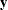dependent variable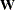diagonal matrix of observation weights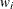weight for the ith observation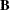B-spline basis for the independent variablenonnegative smoothing parameter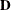difference matrix, penalizes lack of smoothness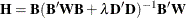hat matrix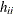ith diagonal element of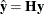penalized B-spline transformation of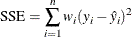error sum of squares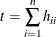trace of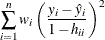CV - cross validation criterion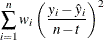GCV - generalized cross validation criterion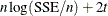AIC - Akaike’s information criterionAICC - corrected AIC (default)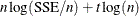SBC - Schwarz’s Bayesian criterion

For more information about constructing the B-spline basis, see SPLINE and MSPLINE Transformations and the section Using Splines and Knots. The nonzero elements of, order 1 are (1 –1), order 2 are (1 –2 1), order 3 (the default) are (1 –3 3 –1), order 4 are (1 –4 6 –4 1), and so on. The nonzero elements for each order are made from the nonzero elements from the preceding order by subtraction: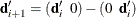. Within an order, the first nonzero element of row i is in column i—that is, each row ofis made from the preceding row by shifting the nonzero elements to the right one position. For example, with k = 4 knots, order o = 3, and degree d = 3,is the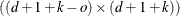matrix: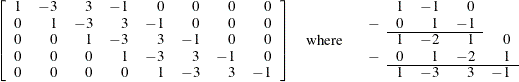The trace of the hat matrix,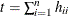, provides an estimate of the number of parameters needed to find the transformation and is used in df calculations. Note, however, that in some cases, particularly with error-free or nearly error-free data, this value can be much larger than you might expect. You might be able to directly create a function by using SPLINE or BSPLINE with many fewer parameters that fits essentially just as well as the penalized B-spline function.

By default with PBSPLINE, a cubic spline is fit with 100 evenly spaced knots, three evenly spaced exterior knots, and a difference matrix of order three. Options are specified as follows: PBSPLINE(x / DEGREE=3 NKNOTS=100 EVENLY=3 PARAMETER=3). By default, PROC TRANSREG searches for an optimal lambda in the range 0 to 1E6 by using parabolic interpolation and Brent’s (Brent, 1973; Press et al., 1989) method. Alternatively, you can specify a lambda range or a list of lambdas by using the LAMBDA= option. Be aware, however, LAMBDA=0 and values near zero might cause numerical problems including floating point errors. Also be aware that larger lambdas might cause numerical problems—for example, the error sum of squares for the model,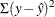, might be greater than the total sum of squares,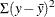—implying that the model with the transformation fits less well than simply predicting by using the mean. When this happens, you will see this message: ERROR: Degenerate transformation with PBSPLINE.

You can fit a single curve through a scatter plot (yx) as follows:

model identity(y) = pbspline(x);


Alternatively, you can fit multiple curves through a scatter plot, one for each level of Group, as follows:

model identity(y) = class(group / zero=none) * pbspline(x);


There are several options for how the smoothing parameter,, is chosen. Usually, you do not specify the smoothing parameter,, and you let PROC TRANSREG choosefor you by minimizing one of the information or cross validation criteria. By default, PROC TRANSREG first considers ranges defined by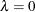and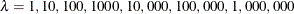. If it finds a range that includes the minimum, it stops and does not consider largervalues. Then it performs further searches in that range. For example, if the initial evaluations at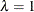and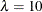show that there is at least a local minimum in the range 0 to 10, then larger values are not considered. Note that the zero smoothing case,, provides a boundary on the range even though the criterion is not evaluated at. The criterion is not evaluated atunless LAMBDA=0 is the only value specified. Also note that the default approach is not the same as specifying the options LAMBDA=0 1E6 RANGE. When a range of values is specified, along with the RANGE t-option, PROC TRANSREG does not try to find smaller ranges based on powers of 10.

PROC TRANSREG avoids evaluating the criterion for LAMBDA= values at or near zero unless you force it to consider them. This is because zero smoothing is rarely interesting and the results are numerically unstable. Values ofat or near zero often result in predicted values that are far outside the range of the data, particularly with interpolation and x values that do not appear in the data set. Also, zero smoothing is prone to numerical problems including floating point errors. This is particularly true when there is a small number of observations, a large number of knots, a high degree, or a perfect or near perfect fit. If you force PROC TRANSREG to evaluate the criterion at or near, you can easily get bad results.

Note that when some observations appear more than once, such as when you have the kind of data where you can use a FREQ statement, then you should consider directly specifying lambda based on a preliminary analysis, ignoring the frequencies. Alternatively, specify a range ofvalues, such as LAMBDA=0.1 1E6 RANGE, that steersaway from values near zero. With the default lambda list, a cross validation criterion does not perform well in choosing a smoothing parameter with replicated data. Leaving one observation out of the computations changes the frequency for that observation from one positive integer to the next smaller positive integer, so in some sense, the point corresponding to that observation is never really left out of any computations. The resulting fit will be undersmoothed unless you specify a larger.